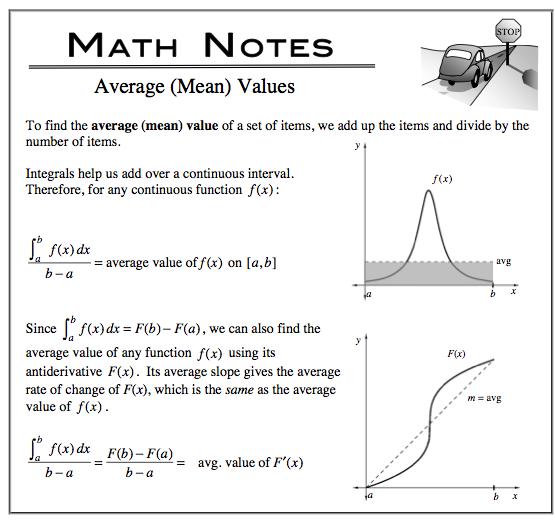### Home > CALC > Chapter 7 > Lesson 7.3.4 > Problem7-146

7-146.

In order to find the average value of a function, sometimes it makes sense to integrate while other times we find the slope of a secant line. When do we use each strategy?

When trying to find the average value of $f(x)$, the strategy depends on what function is given: $f(x)$ or $F(x)$.